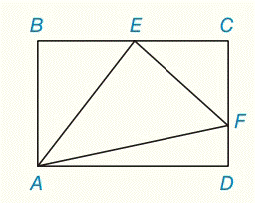Chapter 5.CR, Problem 33CRElementary Geometry For College St...

7th Edition
Alexander + 2 others
ISBN: 9781337614085

Solutions

Chapter
SectionElementary Geometry For College St...

7th Edition
Alexander + 2 others
ISBN: 9781337614085
Textbook Problem

Given: A B C D ​ ​ ​       is     a     rectangle E is the midpoint of  B C ¯ A B = 16 , C F = 9 , A D = 24 Find: A E , E F , A F , m ∠ A E FTo determine

To find:

AE, EF, AF, mAEF.

Explanation

Given:

Calculation:

From ΔBAE

Let us first take the triangle ΔABC

By Pythagoras theorem,

AE2=BA2+BE2AE2=162+122AE2=256+144AE2=400AE=400AE=20

To find EF, consider the triangle ΔECF

By Pythagoras theorem,

EF2=EC2+CF2EF2=122+92EF2=144+81EF2=225EF=225EF=15

By Pythagoras theorem,

Still sussing out bartleby?

Check out a sample textbook solution.

See a sample solution

The Solution to Your Study Problems

Bartleby provides explanations to thousands of textbook problems written by our experts, many with advanced degrees!

Get Started

Evaluate limx02x12x+1x.

Single Variable Calculus

In Exercises 1728, use the logarithm identities to obtain the missing quantity.

Finite Mathematics and Applied Calculus (MindTap Course List)

Show that sin-1(tanh x) = tan1(sinh x).

Single Variable Calculus: Early Transcendentals, Volume I

Find the derivative of the function. y = esin 2x + sin(e2x)

Single Variable Calculus: Early Transcendentals

True or False: If R is a rectangular region in the plane, then

Study Guide for Stewart's Multivariable Calculus, 8th